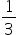Mathematics
Easy

Question

# The unit fraction of the whole number 3 is _____________.

## The correct answer is:### to find - the unit fraction of the whole number 3The unit fraction is a fraction with 1 as the numerator and the integer as denominator. In the whole number 3, the unit fraction is.

Therefore, the unit fraction for the whole number 3 is.

### Related Questions to study#### With Turito Foundation.#### Get an Expert Advice From Turito.